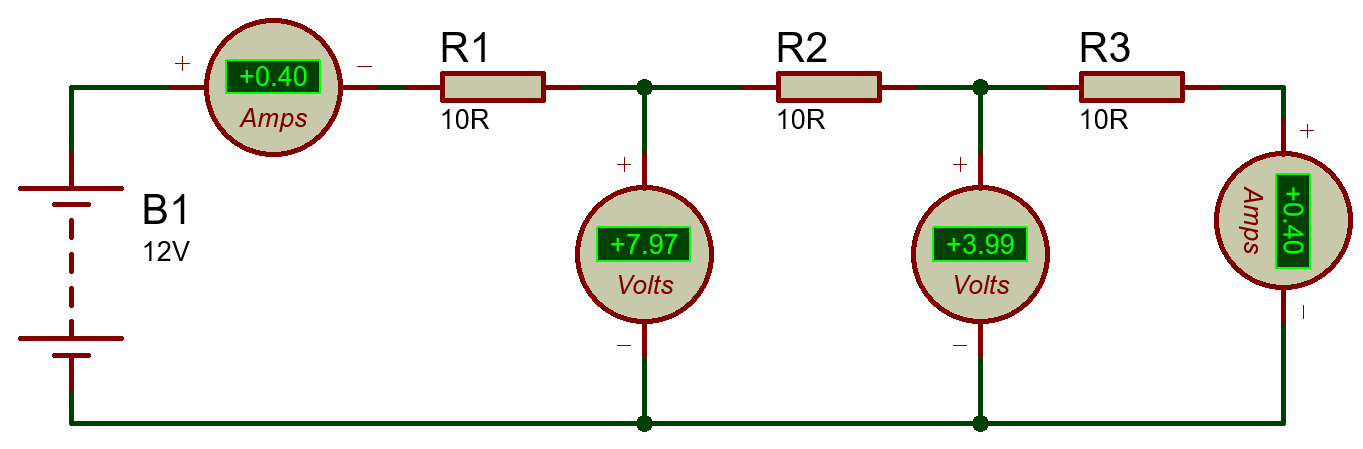## Resistor | Types of Resistor | Resistance calculation using multimeter

Resistor

It is a Two-terminal electrical/electronic component that has the nature of the Resistance. A Resistor is a purely electronic/electrical resistive component that opposes the flow of electrons. The measure of voltage across the resistor’s terminals is called a “voltage drop” across the resistor. Voltage drop is proportional to the resistance value, If there is any change in resistance value then the voltage drop also changes

##RESISTORRESISTOR-SYMBOL                        V = IR (Ohm’s Law)I=V/R

NOTE: So In any design, these are used to voltage, manipulation to get the required rated voltage.

ØIt is a passive device
ØIt has no polarities
ØUnits: ohm (Ω)

Features

## Type: Carbon Film Leaded resistorThe most economic industrial investmentStandard tolerance: +/-5% Excellent long term stabilityTermination: Standard solder-plated copper lead

Resistance Calculation:

##Resistor-Series connection: Voltage vs Current

Parallel Resistance:
• In parallel connection total resistance will be reduced
• We observe the same voltage drop-in each Resistor
• Current will be divide across each resistor – Total current  is equal to sum of current flow  across each resistorResistor-Parallel connection

## Digital potentiometerSMD ResistorArduinoGPSTrim-PotPower resistor                        >>> HOME-TechnoElectronics44>>>

1.2.